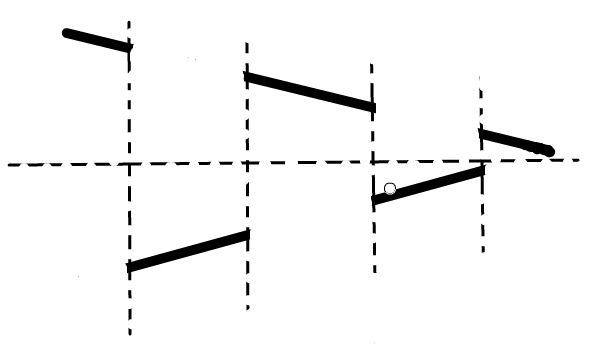1月25日到2月1日，在北京举行了ICPC Camp 2016。我校4支队伍参加了这次Camp。

## Day4. 1.28

### F. Similar Subsequence

dp

#### 做法### G. Random Arithmetic

dp + 数学

#### 做法

$$\sum\limits_{j = 1}^n p[j]$$

$$\sum\limits_{|S| = j}$$

$$\prod\limits_{a[i] \in S} a[i]$$

### H. Optimal BST

dp优化——决策单调性

#### 题目大意

$OPT$的定义为：$OPT[i][j] = min(OPT[i][k] + OPT[k + 1][j] + \sum\limits_{t = i}^ja[t])$